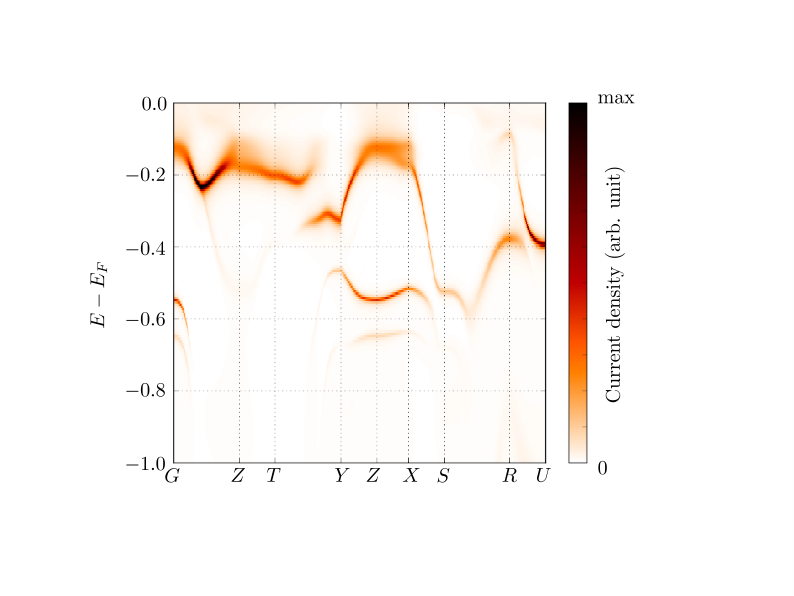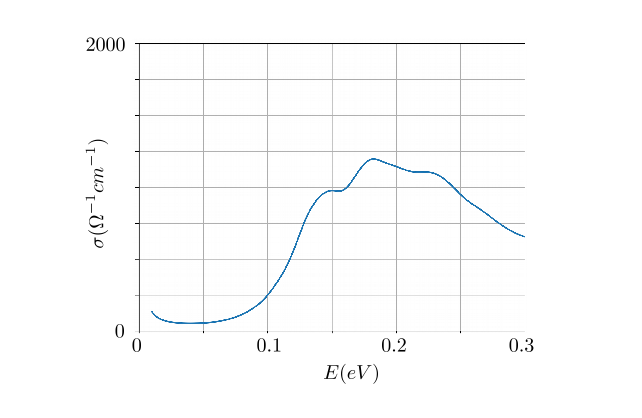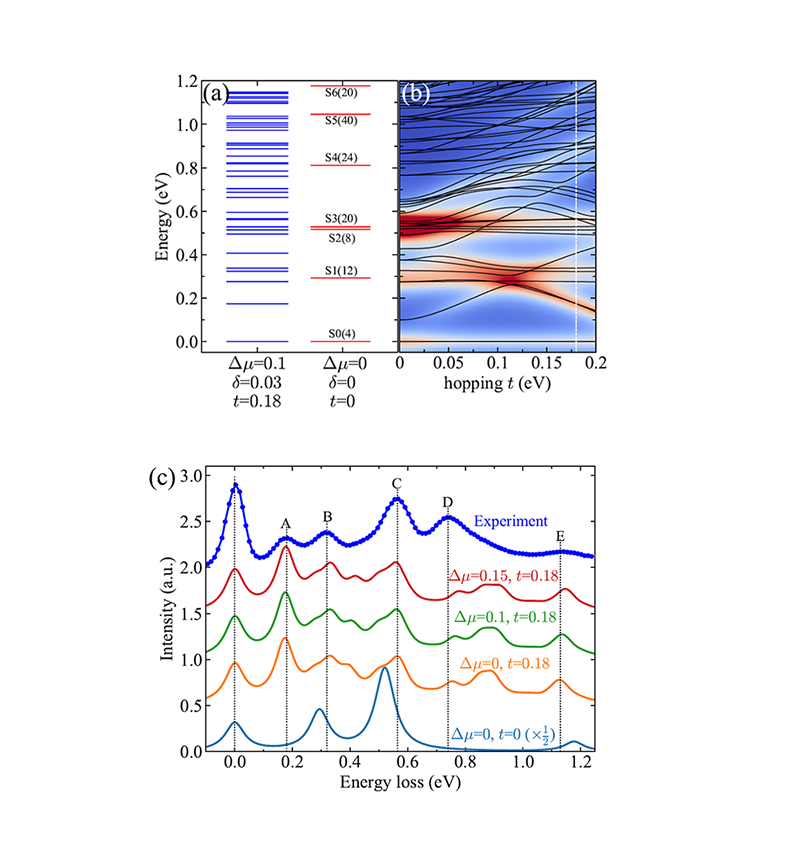research

## Theoretical Spectroscopy

The macroscopic properties of materials are governed by the dynamics of electrons and nuclei. In order to control or make use of the novel physics in various materials it is important to understand these dynamics in detail. Experimentally, one can measure the response of electrons and nuclei in materials to the interaction with probe-particles including electrons and light. Theoretically, it is now possible to calculate the response numerically from first-principles combined with DMFT and its extensions - using the powerful post-processing tools for theoretical spectroscopies in Comsuite. The latter is a fast and flexible approach to the dynamics of electrons in materials and also helps to analyze and explain experimental data by direct comparison between experimental and theoretical spectroscopies. We currently have three theoretical spectroscopy codes: Angle-resolved Photoemission Spectroscopy (ARPES), transport (optical conductivity and Seebeck coefficient), and RIXS.

ARPES: ARPES is a direct experimental technique to observe the electron dynamics in materials by measuring the photoemitted electron current from a sample at various angles. The energy of the incident photon is tuned to excite a valence electron at a reciprocal space point. Theoretically, the photoemitted current can be interpreted as the electron density of states at a reciprocal space point (spectral function) weighted by the light-electron matrix elements. By using the spectral function and matrix elements corresponding to the experimental setup, our ARPES code calculates the spin-polarized photoemitted electron current at various angles.Example of the simulated ARPES on FeSb2.

Transport: With the Comsuite transport codes we can numerically compute the optical conductivity and the Seebeck coefficient by using the electron density of states in reciprocal space (spectral function) and the light-electron matrix elements corresponding to the experimental setup. The optical conductivity is the generalization of the electric conductivity: it measures the linear response of the current density to a frequency-dependent (instead of a static or very slowly varying) electric field. Experimentally, electron-hole excitations are induced in the material by light in optical frequency ranges, and the optical conductivity can be determined via the reflectance (or transmission) of the (very thin) sample—the result of light-emitting particle-hole recombination processes. The Seebeck coefficient measures the electric field that is generated by an applied temperature gradient.Example of the simulated optical conductivity on FeSb2.

RIXS: Resonant Inelastic X-ray Scattering (RIXS) is a photon-in photon-out spectroscopic method to measure the elementary excitations of materials. The energy of the incident photon is tuned at a specific resonant edge to excite a core electron to the valence bands. After a very short time, the core-hole is refilled by a valence electron and a photon is emitted. By measuring the change of energy, momentum and polarization of the scattered photon, one can obtain detailed information on the nature of the underlying excitations in the material. We developed an open source EDRIXS code (see GitHub link)  to simulate the cross-section of RIXS on strongly correlated materials. It exactly diagonalizes (ED) Hamiltonians of a single-atom model, small cluster model or  Anderson impurity model, where the parameters are extracted from a DFT or DFT+DMFT calculation, to get the ground states and uses the Lanczos method to get the excitation spectrum. The core-hole degrees of freedom and the core-hole potential are explicitly included at the atomic level to take care of the large core-hole effects.Examples of the simulated RIXS spectra on Ba5AlIr2O11 at the L3 edge of Ir (2pà 5d), pictures are from Phys. Rev. Lett. 122, 106401 (2019)